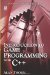# 3.7 Square Numbers and Square Roots

## 3.7 Square Numbers and Square Roots

Subsequent sections focus on examining trigonometry and the importance of right triangles. However, before proceeding we should investigate square numbers and square roots.

Square numbers are the results of numbers that are multiplied by themselves, such as 1 × 1, 2 × 2, 3 × 3, 4 × 4, and so on.The first few square numbers are:

• 1 × 1 = 1

• 2 × 2 = 4

• 3 × 3 = 9

• 4 × 4 = 16

• 5 × 5 = 25

• 6 × 6 = 36

• 7 × 7 = 49

• 8 × 8 = 64

The square roots of those numbers refer to the number which when multiplied by itself would equal that result. So the square root of 4 is 2 because 22 = 4. When a number is squared, we do not typically write, say, 4×4. We saw in an earlier chapter that we use an exponent, such as 42.

Similarly, there is a notation for writing square roots. For this, we use the radical symbol. The number written underneath the radical is called the radicand. An example follows.

Figure 3.15Introduction to Game Programming with C++ (Wordware Game Developers Library)
ISBN: 1598220322
EAN: 2147483647
Year: 2007
Pages: 225
Authors: Alan Thorn

Similar book on Amazon

flylib.com © 2008-2017.
If you may any questions please contact us: flylib@qtcs.net